## RD Sharma Class 10 Solutions Chapter 3 Pair of Linear Equations in Two Variables Ex 3.6

These Solutions are part of RD Sharma Class 10 Solutions. Here we have given RD Sharma Class 10 Solutions Chapter 3 Pair of Linear Equations in Two Variables Ex 3.6

Other Exercises

Question 1.
5 pens and 6 pencils together cost ₹ 9 and 3 pens and 2 pencils cost ₹ 5. Find the cost of 1 pen and 1 pencil. (C.B.S.E. 1991)
Solution:
Let cost of 1 pen = ₹ x
and cost of 1 pencil = ₹ y
According to the conditions,
5x + 6y = 9 ….(i)
3x + 2y = 5 …(ii)
Multiplying (i) by 1 and (ii) by 3, we get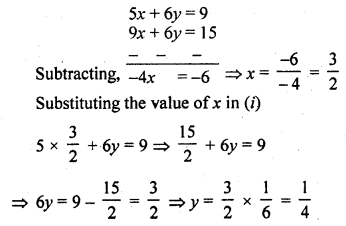Cost of one pen = ₹ $$\frac { 3 }{ 2 }$$
and cost of one pencil = ₹ $$\frac { 1 }{ 4 }$$

Question 2.
7 audio cassettes and 3 video cassettes cost ₹ 1110, while 5 audio cassettes and 4 video cassettes cost ₹ 1350. Find the cost of an audio cassette and a video cassette. (C.B.S.E. 1992)
Solution:
Let the cost of 1 audio cassette = ₹ x
and cost of 1 video cassette = ₹ y
According to the condition,
7x + 3y= 1110 ….(i)
5x + 4y = 1350 ….(ii)
Multiplying (i) by 4 and (ii) by 3,Question 3.
Reena has pens and pencils which together are 40 in number. If she has 5 more pencils and 5 less pens, then number of pencils would become 4 times the number of pens. Find,.the original number of pens and pencils. (C.B.S.E 1992C)
Solution:
Let number of pens = x
and number of pencils = y
x + y = 40 ….(i)
In second case,
number of pens = x – 5
and number of pencils = y + 5
(y + 5) = 4 (x – 5) => y + 5 = 4x – 20
4x – y = 5 + 20 => 4x – y = 25 ….(ii)
5x = 65 => x = 13 [From (i) ]
13 + y = 40 => y = 40 – 13 = 27
Hence number of pens = 13
and number of pencils = 27

Question 4.
4 tables and 3 chairs, together, cost ₹ 2,250 and 3 tables and 4 chairs cost ₹ 1950. Find the cost of 2 chairs and 1 table.
Solution:
Let cost of 1 table = ₹ x
and cost of 1 chair = ₹ y
According to the conditions,
4x + 3y = 2250 ….(i)
3x + 4y= 1950 ….(ii)
Multiplying (i) by 4 and (ii) by 3, we get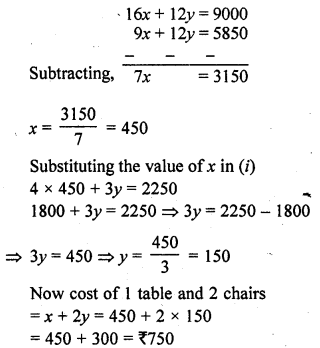Question 5.
3 bags and 4 pens together cost ₹ 257 whereas 4 bags and 3 pens together cost ₹ 324. Find the total cost of 1 bag and 10 pens. (C.B.S.E. 1996)
Solution:
Let cost of 1 bag = ₹ x
and cost of 1 pen = ₹ y
According to the conditions,
3x + 4y = 257 ….(i)
4x + 3y = 324 ….(ii)
Multiplying (i) by 3 and (ii) by 4, we get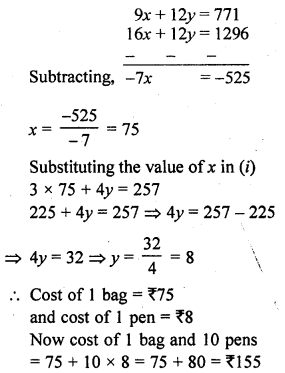Question 6.
5 books and 7 pens together cost ₹ 79 whereas 7 books and 5 pens together cost ₹ 77. Find the cost of 1 book and 2 pens. (C.B.S.E. 1996)
Solution:
Let the cost 1 book = ₹ x
and cost of 1 pen = ₹ y
Now according to the conditions,
5x + 7y = 79 ….(i)
7x + 5y = 77 ….(ii)
Multiplying (i) by 5 and (ii) by 7, we get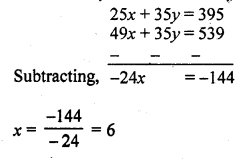Substituting the value of x in (i)
5 x 6 + 7y = 79
=> 30 + 7y = 79
=> 7y = 79 – 30 = 49
y = 7
Cost of 1 book and 2 pens = 6 + 2 x 7 = 6 + 14 = 20

Question 7.
Jamila sold a table and a chair for ₹ 1050, thereby making a profit of 10% on a table and 25% on the chair. If she had taken profit of 25% on the table and 10% on the chair she would have got ₹ 1065. Find the cost price of each. [NCERT Exemplar]
Solution:
Let the cost price of the table be ₹ x
and the cost price of the chair by ₹ y.
The selling price of the table, when it is sold at a profit of 10%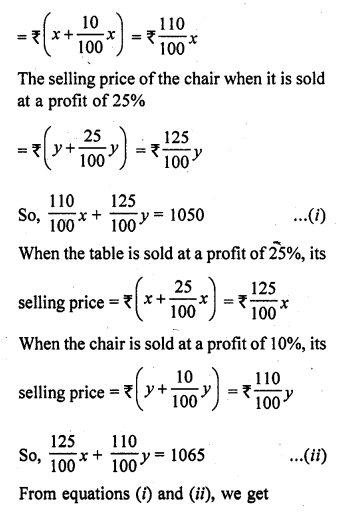110x + 125y = 105000
and 125x + 110y = 106500
On adding and subtracting these equations, we get
235x + 235y = 211500
and 15x – 15y= 1500
i.e., x + y = 900 …(iii)
and x – y = 100 …(iv)
Solving equation (iii) and (iv), we get
2x = 1000
x = 500
500 + y = 900
=> y = 900 – 500
y = 400
x = 500, y = 400
So, the cost price of the table is ₹ 500 and the cost price of the chair is ₹ 400.

Question 8.
Susan invested certain amount of money in two schemes A and B, which offer interest at the rate of 8% per annum and 9% per annum, respectively. She received ₹ 1860 as annual interest. However, had she interchanged the amount of investment in the two schemes, she would have received 720 more as annual interest. How much money did she invest in each scheme?
[NCERT Exemplar]
Solution:
Let the amount of investments in schemes A and B be ₹ x and ₹ y, respectively.
Case I:
Interest at the rate of 8% per annum on scheme A + Interest at the rate of 9% per annum on scheme B = Total amount received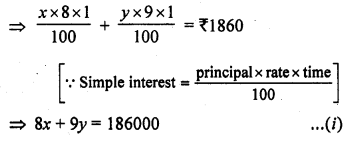Case II:
Interest at the rate of 9% per annum on scheme A + Interest at the rate of 8% per annum on scheme B = ₹ 20 more as annual interest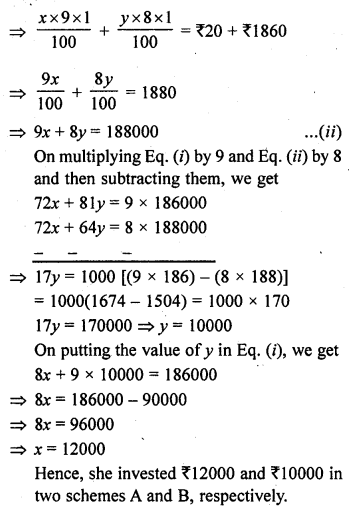Question 9.
The coach of a cricket team buys 7 bats and 6 balls for ₹ 3800. Later, he buys 3 bats and 5 balls for ₹ 1750. Find the cost of each bat and each ball.
Solution:
Let cost of 1 bat = ₹ x
and cost of 1 ball = ₹ y
According to the conditions,
7x + 6y = 3800 ….(i)
3x + 5y = 1750 ….(ii)
Multiplying (i) by 5 and (ii) by 6, we get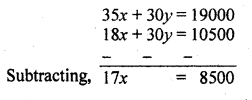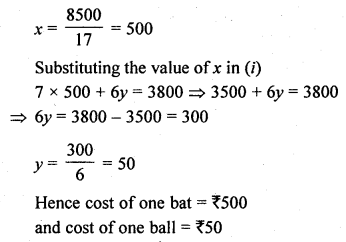Question 10.
A lending library has a fixed charge for the first three days and an additional charge for each day thereafter. Saritha paid ₹ 27 for a book kept for seven days, while Susy paid ₹ 21 for the book she kept for five days. Find the fixed charge and the charge for each extra day;
Solution:
Let the fixed charge for the book = ₹ x
and let extra charge for each day = ₹ y
According to the given conditions,
x + 4y = 27 ….(i)
x + 2y = 21 ….(ii)
Subtracting,
2y = 6 => y = 3
Substituting the value of y in (i)
x + 4 x 3 = 27
=> x + 12 = 27
=> x = 27 – 12 = 15
Amount of fixed charge = ₹ 15
and charges for each extra day = ₹ 3

Question 11.
The cost of 4 pens and 4 pencil boxes is ₹ 100. Three times the cost of a pen is ₹ 15 more than the cost of a pencil box. Form the pair of linear equations for the above situation. Find the cost of a pen and pencil box. [NCERT Exemplar]
Solution:
Let the cost of a pen be ₹ x and the cost of a pencil box be ₹ y.
Then, by given condition,
4x + 4y = 100 => x + y = 25 …(i)
and 3x = y + 15
=> 3x – y = 15 …(ii)
On adding Eqs. (i) and (ii), we get
4x = 40 => x = 10
By substituting x = 10, in Eq. (i) we get
y = 25 – 10 = 15
Hence, the cost of a pen and a pencil box are ₹ 10 and ₹ 15, respectively.

Question 12.
One says, “Give me a hundred, friend! I shall then become twice as rich as you.” The other replies, “If you give me ten, I shall be six times as rich as you.” Tell me what is the amount of their respective capital?
Solution:
Let the amount of first person = ₹ x
and amount of second = ₹ y
According to the first condition,
x + 100 = 2 (y- 100)
=> x + 100 = 2y – 200
=> x – 2y = -200 – 100
=> x – 2y = -300 …….(i)
According to the second condition,
6(x – 10) = (y + 10)
6x – 60 = y + 10
6x – y = 10 + 60
6x – y = 70 ….(ii)
Multiplying (i) by 1 and (ii) by 2, we get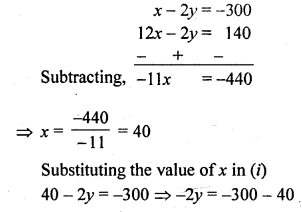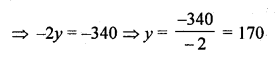Hence first person has money = ₹ 40 and second person has = ₹ 170

Question 13.
A and B each have a certain number of mangoes. A says to B, “if you give 30 of your mangoes, I will have twice as many as left with you.” B replies, “if you give me 10, I will have thrice as many left with you.” How many mangoes does each have ?
Solution:
Let A has mangoes = x
and B has mangoes = y
According to the first condition,
x + 30 = 2 (y – 30)
x + 30 = 2y – 60
x – 2y = -60 – 30
=> x – 2y = -90 ….(i)
and according to the second condition
3 (x – 10) = (y + 10)
=> 3x – 30 = y + 10
=> 3x – y = 10 + 30
=> 3x – y = 40 ….(ii)
From (i) x = -90 + 2y Substituting in (i)
3 (-90 + 2y) – y = 40
– 270 + 6y – y = 40
=> 5y = 40 + 270 = 310
=> y = 62
and x = – 90 + 2y = – 90 + 2 x 62 = 124 – 90 = 34
A has mangoes = 34
and B has mangoes = 62

Question 14.
Vijay had some bananas, and he divided them into two lots A and B. He sold first lot at the rate of ₹ 2 for 3 bananas and the second lot at the rate of ₹ 1 per banana and got a total of ₹ 400. If he had sold the first lot at the rate of ₹ 1 per banana and the second lot at the rate of ₹ 4 per five bananas, his total collection would have been ₹ 460. Find the total number of bananas he had. [NCERT Exemplar]
Solution:
Let the number of bananas in lots A and B be x and y, respectively.
Case I:
Cost of the first lot at the rate of ₹ 2 for 3 bananas + Cost of the second lot at the rate of ₹ 1 per banana = Amount received
=> $$\frac { 2 }{ 3 }$$ x + y = 400
=> 2x + 3y= 1200 …(i)
Case II:
Cost of the first lot at the rate of ₹ 1 per banana + Cost of the second lot at the rate of ₹ 4 for 5 bananas = Amount received
=> x + $$\frac { 4 }{ 5 }$$ y = 460
=> 5x + 4y = 2300 …(ii)
On multiplying in the Eq. (i) by 4 and Eq. (ii) by 3 and then subtracting them, we get,Now, putting the value of x in Eq. (i), we get,
2 x 300 + 3y = 1200
=> 600 + 3y = 1200
=> 3y = 1200 – 600
=> 3y = 600
=> y = 200
Total number of bananas = Number of bananas in lot A + Number of bananas in lot B
= x + y
= 300 + 200 = 500

Question 15.
On selling a T.V. at 5% gain and a fridge at 10% gain, a shopkeeper gains ₹ 2000. But if he sells the T.V. at 10% gain and the fridge at 5% loss. He gains ₹ 1500 on the transaction. Find the actual prices of T.V. and fridge.
Solution:
Let the price of T.V. = ₹ x
and price of Fridge = ₹ y
According to first condition,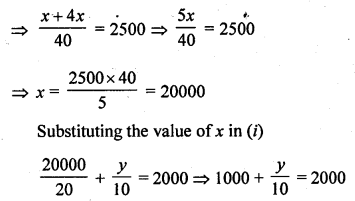$$\frac { y }{ 10 }$$ = 2000 – 1000 = 1000
=> y = 10 x 1000 = 10000
Hence price of T.V. = ₹ 20000 and of fridge = ₹ 10000

Hope given RD Sharma Class 10 Solutions Chapter 3 Pair of Linear Equations in Two Variables Ex 3.6 are helpful to complete your math homework.

If you have any doubts, please comment below. Learn Insta try to provide online math tutoring for you.